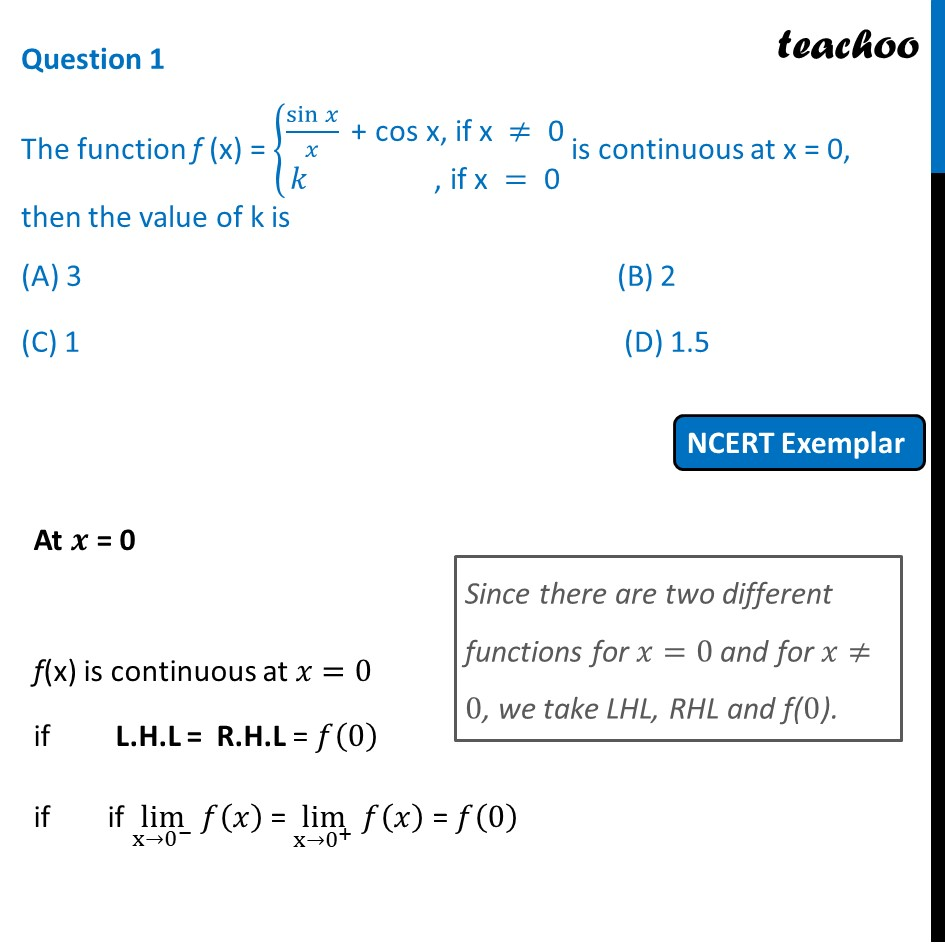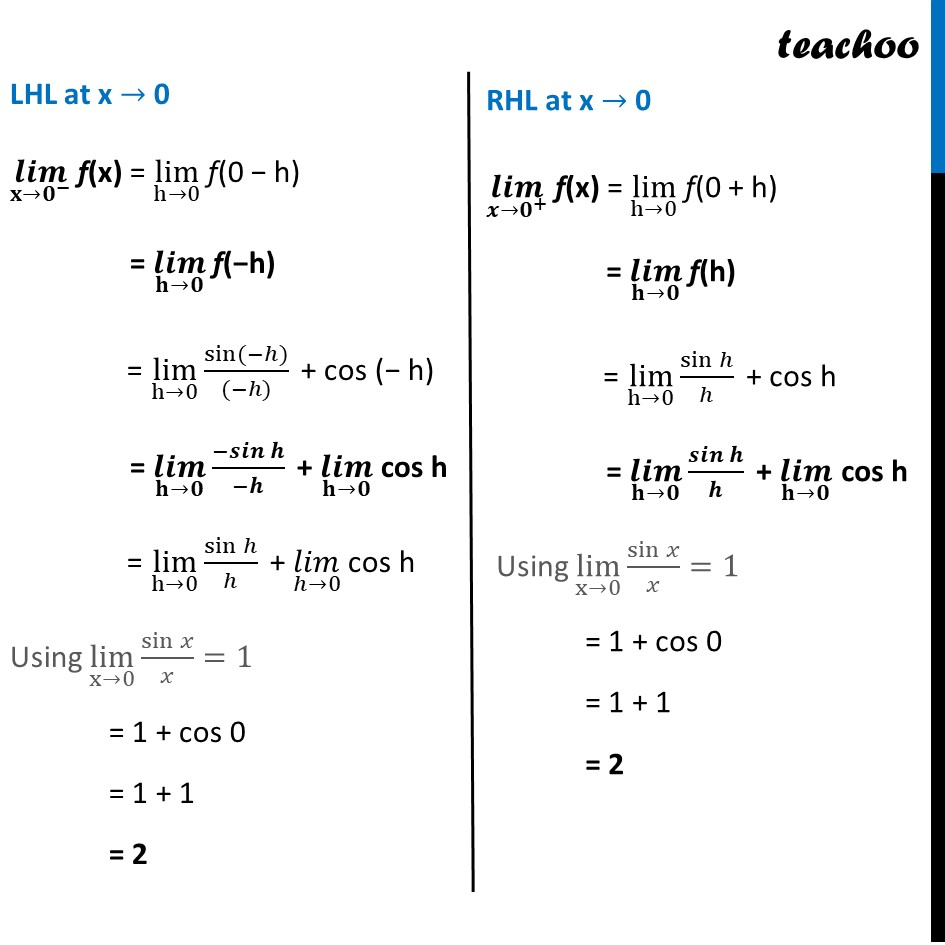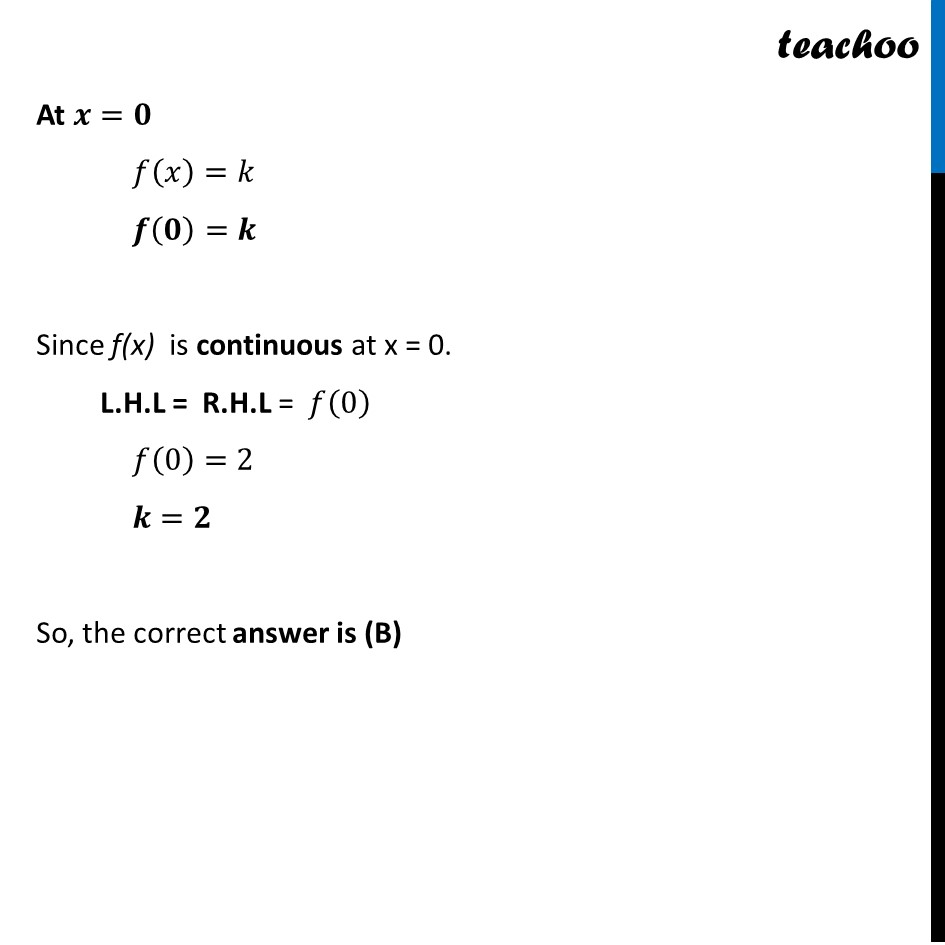NCERT Exemplar - MCQs

Chapter 5 Class 12 Continuity and Differentiability
Serial order wise

## (D) 1.5

This question is similar to Ex 5.1, 18 - Chapter 5 Class 12 - Continuity and DifferentiabilityLearn in your speed, with individual attention - Teachoo Maths 1-on-1 Class

### Transcript

Question 1 The function f (x) = {■8(sin⁡𝑥/𝑥 " + cos x, if x " ≠" 0" @𝑘 ", if x " =" 0" )┤ is continuous at x = 0, then the value of k is (A) 3 (B) 2 (C) 1 (D) 1.5 At 𝒙 = 0 f(x) is continuous at 𝑥=0 if L.H.L = R.H.L = 𝑓(0) if if lim┬(x→0^− ) 𝑓(𝑥) = lim┬(x→0^+ ) 𝑓(𝑥) = 𝑓(0) LHL at x → 0 (𝒍𝒊𝒎)┬(𝐱→𝟎^− ) f(x) = lim┬(h→0) f(0 − h) = (𝒍𝒊𝒎)┬(𝐡→𝟎) f(−h) = lim┬(h→0) sin⁡〖(−ℎ)〗/((−ℎ)) " + cos (− h)" = (𝒍𝒊𝒎)┬(𝐡→𝟎) 〖−𝒔𝒊𝒏〗⁡𝒉/(−𝒉) " +" (𝒍𝒊𝒎)┬(𝐡→𝟎) " cos h" = lim┬(h→0) sin⁡ℎ/ℎ " + " (𝑙𝑖𝑚)┬(ℎ→0) " cos h" Using lim┬(x→0) sin⁡𝑥/𝑥=1 = 1 + "cos 0" = 1 + 1 = 2 RHL at x → 0 (𝒍𝒊𝒎)┬(𝒙→𝟎^+ ) f(x) = lim┬(h→0) f(0 + h) = (𝒍𝒊𝒎)┬(𝐡→𝟎) f(h) = lim┬(h→0) sin⁡ℎ/ℎ " + cos h" = (𝒍𝒊𝒎)┬(𝐡→𝟎) 𝒔𝒊𝒏⁡𝒉/𝒉 " + " (𝒍𝒊𝒎)┬(𝐡→𝟎) " cos h" Using lim┬(x→0) sin⁡𝑥/𝑥=1 = 1 + "cos 0" = 1 + 1 = 2 At 𝒙=𝟎 𝑓(𝑥)=𝑘 𝒇(𝟎)=𝒌 Since f(x) is continuous at x = 0. L.H.L = R.H.L = 𝑓(0) 𝑓(0)=2 𝒌=𝟐 So, the correct answer is (B)